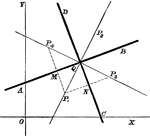### Line Bisectors

Multiple lines showing many bisectors on the coordinate plane.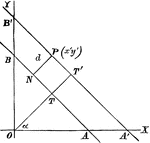### Perpendicular Distance

Finding the perpendicular distance of points whose equation is x cosa + y sina = p.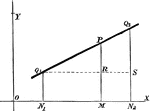### Line Equation

Finding the equation of a line through two given points.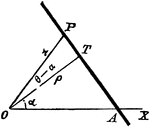### Polar Equation

Finding the polar equation of a straight line.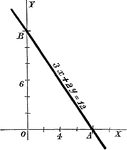### General Form

A line with a standard form equation.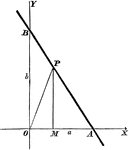### Intercept Form

A line with intercept form equation.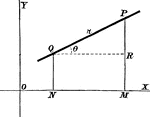### Given Incline

Equation of a line with two given points and a given inclination.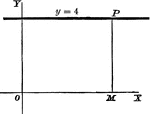### Perpendicular Line

A perpendicular line on a coordinate plane, showing what y the y-coordinate will be.### Straight Line

A positive slope line making a 60 degree angle with the x-axis.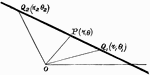### Straight Line

Finding the polar equation of a straight line by passing through two given points.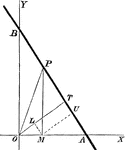### Alternate Method

Another method for using perpendicular form.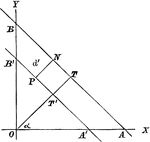### Alternate Form, Perpendicular Distance

An alternate form of the perpendicular distance method.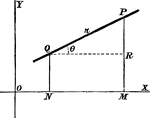### Given Point

Finding the equation of a line drawn through a given point at a given direction.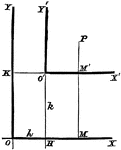### Axes Shift

Any system of coordinate axes to another set which is parallel to the former, but with different origins.